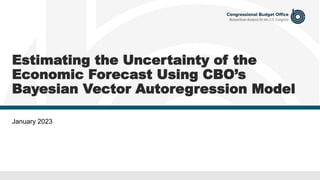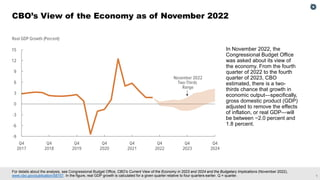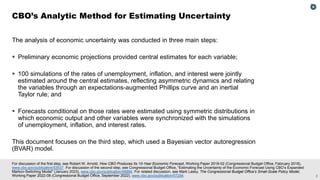Diese Präsentation wurde erfolgreich gemeldet.

# Estimating the Uncertainty of the Economic Forecast Using CBO’s Bayesian Vector Autoregression Model

Anzeige
Anzeige
Anzeige
Anzeige
Anzeige
Anzeige
Anzeige
Anzeige
Anzeige
Anzeige
Anzeige×

1 von 17 Anzeige

# Estimating the Uncertainty of the Economic Forecast Using CBO’s Bayesian Vector Autoregression Model

This slide deck describes how CBO used a Bayesian vector autoregression model to assess the uncertainty of the economic forecast presented in CBO’s Current View of the Economy in 2023 and 2024 and the Budgetary Implications (November 2022).

This slide deck describes how CBO used a Bayesian vector autoregression model to assess the uncertainty of the economic forecast presented in CBO’s Current View of the Economy in 2023 and 2024 and the Budgetary Implications (November 2022).

Anzeige
Anzeige

### Estimating the Uncertainty of the Economic Forecast Using CBO’s Bayesian Vector Autoregression Model

1. 1. Estimating the Uncertainty of the Economic Forecast Using CBO’s Bayesian Vector Autoregression Model January 2023
2. 2. 1 For details about the analysis, see Congressional Budget Office, CBO’s Current View of the Economy in 2023 and 2024 and the Budgetary Implications (November 2022), www.cbo.gov/publication/58757. In the figure, real GDP growth is calculated for a given quarter relative to four quarters earlier. Q = quarter. CBO’s View of the Economy as of November 2022 In November 2022, the Congressional Budget Office was asked about its view of the economy. From the fourth quarter of 2022 to the fourth quarter of 2023, CBO estimated, there is a two- thirds chance that growth in economic output—specifically, gross domestic product (GDP) adjusted to remove the effects of inflation, or real GDP—will be between −2.0 percent and 1.8 percent.
3. 3. 2 For discussion of the first step, see Robert W. Arnold, How CBO Produces Its 10-Year Economic Forecast, Working Paper 2018-02 (Congressional Budget Office, February 2018), www.cbo.gov/publication/53537. For discussion of the second step, see Congressional Budget Office, “Estimating the Uncertainty of the Economic Forecast Using CBO’s Expanded Markov-Switching Model” (January 2023), www.cbo.gov/publication/58884. For related discussion, see Mark Lasky, The Congressional Budget Office’s Small-Scale Policy Model, Working Paper 2022-08 (Congressional Budget Office, September 2022), www.cbo.gov/publication/57254. The analysis of economic uncertainty was conducted in three main steps: ▪ Preliminary economic projections provided central estimates for each variable; ▪ 100 simulations of the rates of unemployment, inflation, and interest were jointly estimated around the central estimates, reflecting asymmetric dynamics and relating the variables through an expectations-augmented Phillips curve and an inertial Taylor rule; and ▪ Forecasts conditional on those rates were estimated using symmetric distributions in which economic output and other variables were synchronized with the simulations of unemployment, inflation, and interest rates. This document focuses on the third step, which used a Bayesian vector autoregression (BVAR) model. CBO’s Analytic Method for Estimating Uncertainty
4. 4. 3 The BVAR model draws on historical correlations between macroeconomic variables to produce conditional forecasts. Key conditions are the simulations of the rates of unemployment, inflation, and interest, which are estimated using an expanded version of CBO’s Markov-switching model with asymmetric dynamics in which the unemployment rate rises rapidly in some periods and falls gradually in others and interest rates do not fall below zero. The projections of economic output and other variables are synchronized—using symmetric distributions—with the simulations of unemployment, inflation, and interest rates. Additional variables that can be simulated with symmetric distributions can be easily incorporated. The historical correlations between macroeconomic variables used in the model may be less predictive of future outcomes in the event of extreme changes in economic conditions. Historical Dynamics Reflected in the Modeling
5. 5. 4 Inputs include central forecasts from CBO’s large-scale macroeconometric model for 26 variables used to analyze effects of economic conditions on the federal budget. Additional inputs are 100 simulations of six variables from CBO’s expanded Markov- switching model: the unemployment rate, two inflation rates, and three interest rates. For each calendar quarter, CBO uses those inputs to the BVAR model to project values for the remaining 20 variables. After 100 simulations are generated, the values are calibrated so that their average equals CBO’s central forecast. The parameters of the model are estimated using data from 1959 through 2022. How CBO Uses the Model
6. 6. 5 The unemployment rate projection is taken as a set of conditions. CBO uses the BVAR model to project the following: ▪ Payroll employment, ▪ The number of people in the labor force, ▪ Hours of work, and ▪ Wages and salaries. Labor Market Variables Projected Using the Model
7. 7. 6 Two rates of inflation are taken as sets of conditions—the overall rate as measured by the personal consumption expenditures price index and that rate excluding food and energy prices. CBO uses the BVAR model to project inflation as measured by: ▪ The GDP price index, ▪ The consumer price index for all urban consumers, ▪ The consumer price index for food at home, and ▪ The consumer price index for medical care. Inflation Rates Projected Using the Model
8. 8. 7 Three interest rates are taken as sets of conditions—the federal funds rate (the rate that financial institutions charge each other for overnight loans of their monetary reserves), the 3-month Treasury bill rate, and the 10-year Treasury note rate. CBO uses the BVAR model to project the following: ▪ The 5-year Treasury note rate, ▪ The corporate Aaa bond rate, and ▪ The corporate Baa bond rate. Interest Rates Projected Using the Model
9. 9. 8 CBO uses the BVAR model to project the following: Output Variables Projected Using the Model ▪ Real GDP ▪ Real personal consumption expenditures ▪ Real nonresidential fixed investment ▪ Real exports ▪ Real imports ▪ Total factor productivity ▪ Real potential GDP ▪ Nominal gross national product ▪ Nominal private nonresidential fixed investment in equipment
10. 10. 9 See Richard K. Crump and others, A Large Bayesian VAR of the United States Economy, Staff Report 976 (Federal Reserve Bank of New York, August 2021), http://newyorkfed.org/research/staff_reports/sr976.html. CBO’s modeling follows this approach closely, and this staff report provides more details than are included here. CBO adapted its approach to conditional forecasting from that used by the staff of the Federal Reserve Bank of New York. Bayesian techniques are particularly well suited to estimating parameters in a large system of equations given a limited amount of data. The modeling is structured so that a projection of a variable at a given point in time is more likely to be influenced by recent data than by older data. The structure prevents the estimation from explaining historical data well but having poor ability to forecast beyond the data used for estimation—which would be the case if the estimation process overfit the parameters. The approach is flexible, and the staff of the Federal Reserve Bank of New York found that it generated reasonable conditional forecasts. How the Model Works
11. 11. 10 CBO used the following equation: yt = c + B1yt - 1 + ⋯ + Bp yt - p + ϵt , ϵt ~N(0,Σ) where ▪ yt is a vector of m economic variables at time t (t = 1, … , T), ▪ Bs (s = 1, … , p) is a (m × m) matrix of parameters of lagged variables, and ▪ ϵt is an error term distributed by a normal distribution with a covariance matrix Σ. The model has many parameters to be estimated when the number of variables is large. Because m equals 26 and p equals 6 in the model, the set of Bs (s = 1, … , p) has 4,056 parameters (26 × 26 × 6). In this case, the traditional vector autoregression techniques are vulnerable to overfitting and tend to show poor out-of-sample forecasting accuracy. A Bayesian procedure addresses the overfitting issue by automatically selecting the degree of shrinkage, using tighter priors when the number of unknown coefficients relative to available data is high and looser priors otherwise. The Bayesian Vector Autoregression Model
12. 12. 11 For discussion of the Minnesota prior, see Robert Litterman, Techniques of Forecasting Using Vector Autoregressions, Working Paper 115 (Federal Reserve of Minneapolis, November 1979), www.minneapolisfed.org/research/working-papers/techniques-of-forecasting-using-vector-autoregressions. CBO used the Minnesota prior, under which each variable follows an independent random walk process with potential drift. The prior sets the mean of each Bs as where (Bs)ij is the row i, column j element of Bs. The Minnesota prior sets tighter distributions for the parameters corresponding to smaller lags. Variances and covariances between elements of B are set as where ▪ λ determines the general tightness of the prior distribution of B, ▪ Σik is the row i, column k element of Σ, and ▪ ψj is an estimate of the variation of variable j in yt. CBO estimated λ using the hierarchical Bayesian approach. The Bayesian Prior Distribution
13. 13. 12 The posterior distribution of the parameters was calculated via Bayes’ rule as p(θ│YT )∝ p(θ)p(YT |θ) where ▪ θ is the vector of the parameters in the BVAR (θ = (c, B1, … , Bp, Σ)); ▪ YT is the vector of the historical values of all the variables or YT = (y1, … , yT); ▪ p(θ) is the prior distribution (the Minnesota prior); and ▪ p(YT |θ) is the likelihood function of the BVAR. CBO used a Markov chain Monte Carlo (MCMC) algorithm to generate the draws of θ (or θ(g) for g = 1, … , G) from the posterior distribution. The Posterior Distribution and Draws of Parameters
14. 14. 13 CBO projected all the variables in the BVAR using Bayesian inference by computing the predictive density, defined as p(yT + 1, … ,yT + h│YT ) = ∫ p(yT + 1, … ,yT + h | YT, θ)p(θ│YT)dθ where h is the forecasting horizon. In practice, the future value is projected for each MCMC draw of the parameters (θ(g)) using the structure of the BVAR. The whole set of projected values is the predictive density. CBO also generated conditional forecasts using conditional predictive densities, defined as p(yT + 1, … ,yT + h│YT, Ch) = ∫ p(yT + 1, … ,yT + h | YT, Ch, θ)p(θ│YT )dθ where Ch is a set of given conditions for a scenario. The conditions can be imposed on any of the variables in the BVAR for any time. Forecasting
15. 15. 14 To implement conditional forecasting, CBO cast the BVAR model into a linear state- space model: where ▪ yt * is conditional on future values of some variables in yt (t = T + 1, … , T + H), ▪ xt is (yt, yt - 1,…, yt – p + 1, c)', ▪ Gt is a matrix identifying the conditioned future values in xt, ▪ F is a matrix representing the dynamics of xt, and ▪ ut = (ϵt, 0, … , 0)'. Then, CBO applied the Kalman filter and smoother to generate conditional forecasts. The approach is equivalent to estimating unobservable variables (or missing values) while treating the conditions as observable variables (or nonmissing values). Conditional Forecasting
16. 16. 15 For discussion of CBO’s historical forecasting errors, see Congressional Budget Office, CBO’s Economic Forecasting Record: 2021 Update (December 2021), www.cbo.gov/publication/57579. The variance of the paths generated by conditional forecasting is related to the way economic conditions have changed over time and to the simulations of the six variables from CBO’s expanded Markov-switching model that are taken as sets of conditions. To create simulations for the purpose of communicating uncertainty about the central estimates in the economic forecast, CBO calibrated their variance. Specifically, CBO used the paths generated by conditional forecasting to create 100 simulations of the 20 variables discussed above (including real GDP growth), which incorporated correlations with the six variables from CBO’s expanded Markov-switching model. The variance of real GDP growth was calibrated by considering alternative ways to average multiple paths to form a single simulation. For this analysis, CBO used the simple average of two paths to form each simulation because the range of the middle two-thirds of the distribution of those simulations matched the middle two-thirds of CBO’s historical forecasting errors over two years. Calibrating the Variance of Simulations
17. 17. 16 This document was prepared to enhance the transparency of CBO’s work and to encourage external review of that work. In keeping with CBO’s mandate to provide objective, impartial analysis, the document makes no recommendations. Byoung Hark Yoo prepared the document with guidance from Sebastien Gay. Robert Arnold, Mark Lasky, and Michael McGrane provided comments. Mark Hadley and Jeffrey Kling reviewed the document. Christine Browne edited it and R. L. Rebach created the graphics. The document is available at www.cbo.gov/publication/58883. CBO seeks feedback to make its work as useful as possible. Please send comments to communications@cbo.gov. About This Document